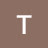Quadratic Formula | Algebra | Chegg TutorsQuadratic Formula | Algebra | Chegg Tutors

The quadratic formula, x = -b ± √b2 – 4ac/2a, is used in algebra to solve quadratic equations (polynomial equations of the second degree). The general form of a quadratic equation is ax2 + bx + c = 0, where x represents a variable, and a, b, and c are constants, with a≠0. A quadratic equation has two solutions, called roots. One simple way to solve quadratic equations is to set each factor equal to zero and then solve for each factor. However, when the quadratic equation is difficult to solve, the quadratic formula is used to find the solutions. Remember that the symbol “±” indicates that both x = -b + √b2 – 4ac/2a and x = -b – √b2 – 4ac/2a are solutions to the quadratic equation.

———-

Algebra tutoring on Chegg Tutors

Learn about Algebra terms like Quadratic Formula on Chegg Tutors. Work with live, online Algebra tutors like Chris W. who can help you at any moment, whether at 2pm or 2am.

Liked the video tutorial? Schedule lessons on-demand or schedule weekly tutoring in advance with tutors like Chris W.

———-

About Chris W., Algebra tutor on Chegg Tutors:

University of Pennsylvania, Class of 2007
Math, Computer Science major

Subjects tutored: Linear Algebra, Pre-Algebra, Applied Mathematics, Calculus, Number Theory, Algebra, Statistics, SSAT (math), Linear Programming, Computer Certification and Training, SAT II Mathematics Level 2, Information Technology, Geometry (College Advanced), SAT (math), PSAT (math), Set Theory, Discrete Math, Basic Math, Trigonometry, ACT (math), LaTeX, SAT II Mathematics Level 1, Numerical Analysis, Web Design, Pre-Calculus, Computer Science, Geometry, Software Engineering, and GRE

TEACHING EXPERIENCE
Over 7 years of experience teaching math at 3 universities and a community college. Courses ranged from Intermediate Algebra to Calculus II and class sizes varied from 2 to over 200 students. Tutoring since 2000 formally and informally, individually and in groups, for courses from Geometry to Differential Equations. Please note that I generally will not be available for audio and video in live lessons but my experience has been that audio and video aren’t really needed.

EXTRACURRICULAR INTERESTS
Hiking, reading, video games, playing the guitar and piano.

Want to book a private lesson with Chris W.?

———-

Like what you see? Subscribe to Chegg’s Youtube Channel:

http://bit.ly/1PwMn3k

———-

Visit Chegg.com for purchasing or renting textbooks, getting homework help, finding an online tutor, applying for scholarships and internships, discovering colleges, and more!

https://chegg.com

———-

Want more from Chegg? Follow Chegg on social media:

http://instagram.com/chegg

algebra tutors,Chegg,Chegg Study,Online Tutoring,Homework Help,Chegg Tutors,Quadratic Formula,Algebra

algebratutors.org

1.Toxical says: